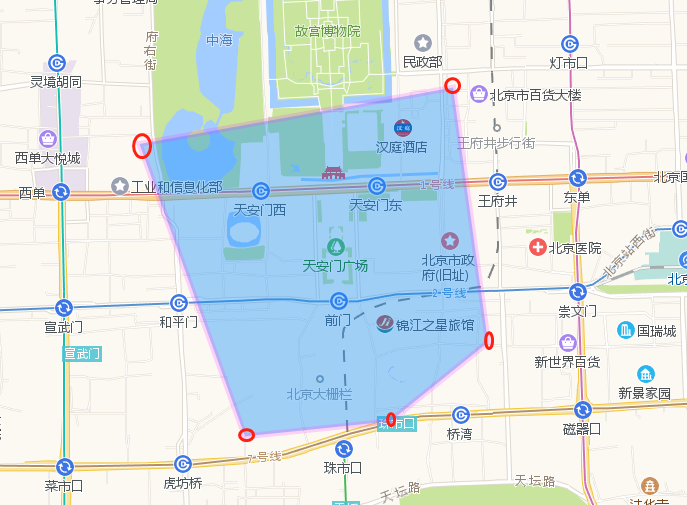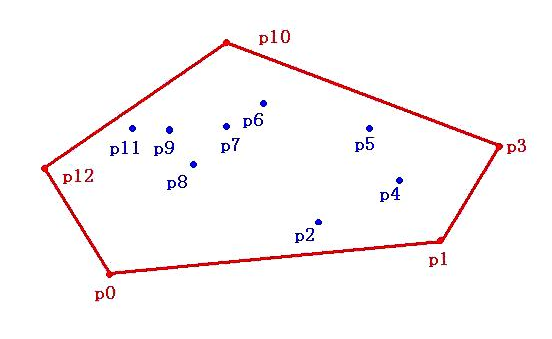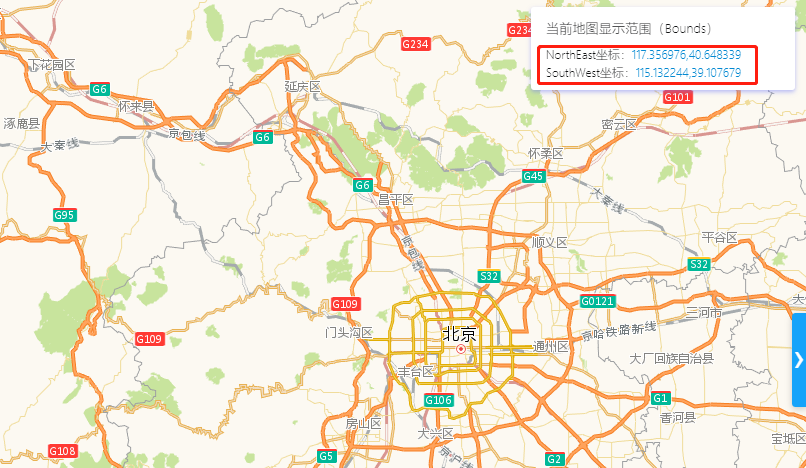使用Path2D和凸包算法实现地理围栏服务 原荐

1.使用Path2D创建一个多边形

Path2D类是java.awt.geom包提供的工具包，可表示任意几何路径的简单而灵活的形状。它可以完全表示PathIterator接口可以迭代的任何路径， 包括其所有段类型和绕组规则，并且它实现了Shape接口的所有基本命中测试方法。

https://lbs.amap.com/api/javascript-api/example/overlayers/polygon-draw-and-edit``````//传参 有序的坐标范围
public static Path2D.Double create(List<PointDouble> polygon) {
//创建path2D对象
Path2D.Double generalPath = new Path2D.Double();
//获取有序坐标范围的第一个坐标
PointDouble first = polygon.get(0);
//通过移动到指定坐标（以双精度指定），将一个点添加到路径中
generalPath.moveTo(first.getX(), first.getY());
//删除有序坐标范围第一个
polygon.remove(0);
//遍历有序坐标范围后面的坐标
for (PointDouble d : polygon) {
// 通过绘制一条从当前坐标到新指定坐标（以双精度指定）的直线，将一个点添加到路径中。
generalPath.lineTo(d.getX(), d.getY());
}
// 将几何多边形封闭
generalPath.lineTo(first.getX(), first.getY());
//关闭路径
generalPath.closePath();
return generalPath;
}

``````

moveTo(double x, double y)

lineTo(double x, double y)

closePath()

2.判断某个坐标是否在Path2D

``````PointDouble point = new PointDouble(116.403322,39.920255);
//生成好的多边形是不是包含某个坐标
path2d.contains(point)``````

contains(double x, double y)

4.使用凸包算法把指定Path2D转换成一块大的覆盖面``````/**
* 凸包算法
*
* @author wangnian
*/
public class ConvexUtil {
/**
* 凸包算法，返回点集合中凸多边形的点集合
*
* @param pointList
* @return
*/
public static List<PointDouble> getConvexPoint(List<PointDouble> pointList) {
//用于计算最终返回结果中是凸包中点的个数
List<PointDouble> resultList = new ArrayList<>();
for (int i = 0; i < pointList.size(); i++) {
for (int j = 0; j < pointList.size(); j++) {
if (j == i) {
//除去选中作为确定直线的第一个点
continue;
}
//存放点到直线距离所使用判断公式的结果
double[] judge = new double[pointList.size()];
for (int k = 0; k < pointList.size(); k++) {
double a = pointList.get(j).getX() - pointList.get(i).getY();
double b = pointList.get(i).getX() - pointList.get(j).getX();
double c = (pointList.get(i).getX()) * (pointList.get(j).getY()) - (pointList.get(i).getY()) * (pointList.get(j).getX());
//根据公式计算具体判断结果
judge[k] = a * (pointList.get(k).getX()) + b * (pointList.get(k).getY()) - c;
}
// 如果点均在直线的一边,则相应的A[i]是凸包中的点
if (JudgeArray(judge)) {
break;
}
}
}
return resultList;
}

/**
* 判断数组中元素是否全部大于等于0或者小于等于0，如果是则返回true，否则返回false
*
* @param Array
* @return
*/
private static boolean JudgeArray(double[] Array) {
boolean judge = false;
int len1 = 0, len2 = 0;
for (int i = 0; i < Array.length; i++) {
if (Array[i] >= 0) {
len1++;
}
}
for (int j = 0; j < Array.length; j++) {
if (Array[j] <= 0) {
len2++;
}
}
if (len1 == Array.length || len2 == Array.length) {
judge = true;
}
return judge;
}

}

``````

5. 根据当前地图窗口查询所有相交Path2D

根据当前地图显示范围获取到northeast东北角和southwest西南角的坐标位置,查询相交的所有Path2D

https://lbs.amap.com/api/javascript-api/example/map/map-bounds/?sug_index=2``````//获取西南的纬度  也就是X
double lng = southwest.getLng();
//获取西南的经度  也就是Y
double lat = southwest.getLat();
//东北角的X减去西南角的X ，得到宽
double w = northeast.getLng() - southwest.getLng();
//东北角的Y减去西南角的Y ，得到高
double h = northeast.getLat() - southwest.getLat();

//判断是否相交
Path2D.intersects(lng, lat, w, h)``````

intersects(double x, double y, double w, double h)